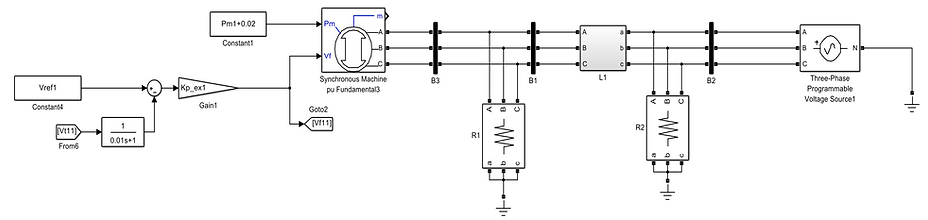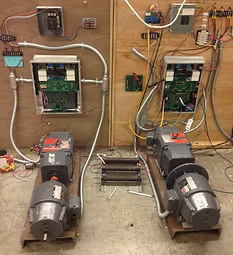top of page
Microgrid

#### Stability Analysis of a Grid-Connected Microgrid

Ph.D. Students: Po-Hsu Huang, Jorge E. Martinez | Advisors: Jimmy C. -H. Peng, and James L. Kirtley, Massachusetts Institute of Technology, USA | Project Duration: 2014 -2016

Microgrids are local networks consisting of generators and loads that are capable of operating in-parallel with, or independently from, the main electric grid. From a technical perspective, the electrical characteristics are similar for microgrids of varying scale, e.g., a building, industrial park, city, or even an entire state. When connected to the main power grid, the issue of angular stability needs to be addressed. Here, the slower responses of traditional synchronous generators are more problematic than those of renewable generations managed by power electronic converters.Play VideoFigure 1. Diagram of a synchronous generator to an infinite bus system

Apart from the immediate issue of voltage stability, the power transfer between a microgrid and the main grid can trigger power oscillations, which are dangerous if not damped properly. Power oscillations tend to occur when the impedance of the transmission line is higher and the power transferred from one side to another is large as shown in Figure 1.

The objective of this research was to develop a configurable testbed that could be customized to analyse the stability of various types of microgrids. The design was carried out in two stages: simulation and experimentation.

Simulation

A microgrid can be modelled as a synchronous generator with a connected load. This is essentially a single-machine-to-an-infinite-bus system, which has been extensively studied in the literature. The procedure for investigating the network stability is summarized as follows:

1. Develop the large-signal model in Simulink (see Figure 2). Network parameters are summarized in Table 1.Figure 2. Large-signal model for simulating a single machine connected to an infinite bus.2.  Determine the equilibrium of normal operations. This can be achieved by doing power flow analysis based on

mathematical derivations of the approximated solutions. Subsequently, compute the exact solutions using trim()

from Maltab toolbox.

3.  Construct a small-signal model using linmod() in Simulink toolbox. The system modes are the eigenvalues of A:
x = A1x + G1z + Bu                                                                                                                                                                                  z = A­2x + G2z
Note that linmod() can also accept Differential Algebra Equations (DAEs).

4.   Execute the sensitivity analysis by perturbing the system parameters and conditions. The simulated results are

summarized in Table 2 and Figure 3.Figure 3. Electromechanical mode deviation due to variations in (a) line impedance; b) inertia constant; and (c) exciter gain.

Using the same procedure, a detailed Simulink model was then constructed to predict the dynamics of the planned experimental conditions. This model captures the non-linear dynamics by including detailed modeling of the power electronics and their corresponding inner control loops.

###### Experimentation - Hardware Setup

Referring to Figure 4, a synchronous machine is connected to the grid through a set of inductors of 11 mH. The autotransformer is configured to step down the grid voltage from 208V to 63V. The reason is because the inertia of the coupled machines (synchronous generator and DC motor) is fairly low, around 0.032 (kg*m2). A DC motor is used to emulate the prime mover. In order to emulate the slow oscillatory power dynamics, the per-unit inertia is found to be around 6.4s. The overall parameters are shown in Table 3.Figure 4. System configuration of the single-machine microgrid.

Table 3: System parameters of the experimental microgridFigure 5. Prototyped controller that emulates the dynamics of different types of synchronous generators.

Table 4: Control parametersThe control algorithm of the synchronous generator is implemented in a controller as shown in Figure 5. The prototyped module consisted of two Microchip processors (dsPIC33EP512GM710). The first processor is used for generating PWM signals to control the exciter as well as the DC motor though the buck converters. Its ADC channels capture the measurements of exciter current, field current, stator voltage/current, active power/reactive power, and armature current. Meanwhile, the second processor collects the measured data from the first one via SPI, and then transmits them to the LabView platform via RS485. The control parameters used in this research are summarized in Table 4.

Experimental Results

Two experimental scenarios were tested. Perturbations were generated by a sudden change in the mechanical power of the generator to trigger the oscillation dynamics. The goal was to mimic an active power imbalance caused by a load change. The experimental results were recorded by LabView, and then compared with the results from the detailed Simulink model. Note that one machine had a better shaft/coupling balance than another such that the results from them were notably different in terms of the waveform quality. Referring to Case S1 in Figure 6, the waveforms after a sudden increase in the mechanical power to 0.3 per unit are similar for those obtained from simulations and experiments. This verifies the validity of the system design. From the small-signal analysis, the frequency of the oscillatory mode is around 0.68 Hz with a damping ratio of 0.53%. A snapshot of the oscilloscope recorded for Case S1 is shown in Figure 7.Figure 6. Comparison of the simulated and experimental results (Case S1).Figure 7. Current and voltage waveforms of the synchronous generator for Case S1.

The results of Case S2 are shown in Figure 8. Here, the machine was subjected to a sudden change in power of 0.5 per unit. It can be seen that the numerical model and the experimental setup matches nicely. Also, the small-signal results confirm that the oscillation is weakly damped at 0.70 Hz with a damping ratio of 0.04%.

To conclude, a higher transmitted power from the microgrid increases the oscillatory frequency and decreases the network damping. Therefore, adequate control settings are required to ensure that the power oscillations are properly damped. Furthermore, inadequate settings could cause controllers to fight among each other --- an emerging stability issue in microgrids.Figure 8. Comparison of the simulated and experimental results (Case S2).

bottom of page